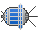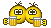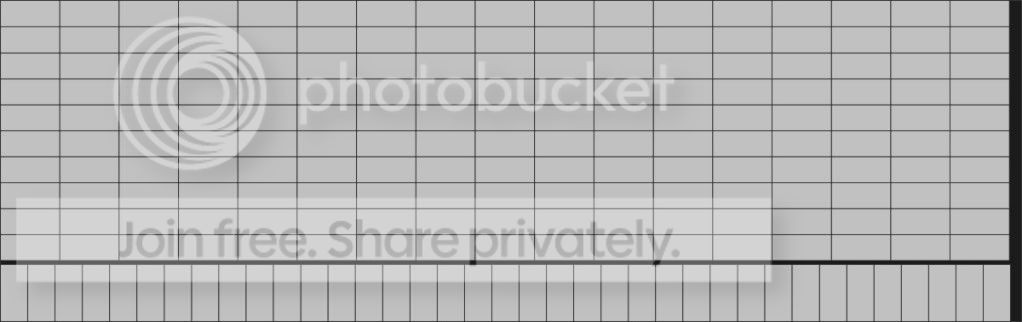# ScienceEasy maths problems I had for an exam, just want to be sure...

#### N_Molson

Donator
As the title says, I recently passed a math exam with some maths questions, and I'm not 100% sure of my answers, though I double checked them...

I guess that someone with good skill at maths can solve them in a few seconds.

PROBLEM 1 :

We know that the area of a (regular) hexagon is : [(3*(sqrt 3))/2]*radius²

We have an hexagonal "M10" brass nut. Dimensions are :

Diameter (maximal diameter) : 18.9 milimeters
Hole diameter : 10 mm
Height : 8 mm

1) Get the exact (no numbers with endless period) volume of the nut, and give an approximation in cubic milimeters (more or less 1 cubic milimeter) of that volume.

2) We know that the molar mass of brass is 8,400 kg (8.4T) /cubic meter. Give the mass of the nut (in grams), more or less 1 gram.

PROBLEM 2 (very easy) :

This one is a part of a physics problem, so the result is essential for later calculations...

We have a flat roof, which dimensions are 8*24.5 meters.

We want to put as much solar panels as possible on it.

The dimensions of a solar panel are 1424 * 655 milimeters.

Obviously you can't cut a solar panel. You can imagine this problem takes place on the Moon if you're addicted to Orbiter.So, if someone can help me to check my answers, you're welcome. That would probably help me to guess if I'll pass the examwoohooor notfacepalm.

:hail:#### Lunar_Lander

##### New member
1)
Well, let's see. First calculate the volume of the nut if it hadn't got a hole. Using your formula:

A_Hexagon=[(3*(sqrt 3))/2]*(18.9mm/2)^2=232 mm^2
V_Hexagon=232 mm^2*8mm=1856 mm^3

Now the hole in the nut is a cylinder, being cut out of the nut. Using V=Pi*r^2*h:

V_Hole=Pi*(5 mm)^2*8mm=628.3 mm^3

V_Nut=V_Hexagon-V_Hole=1856 mm^3-628.3 mm^3=1227.7 mm^3

m_Nut=Rho_Brass*V_Nut=1227.7 mm^3*8400 kg/m^3=10.31 g.

Note though, that 8400 kg/m^3 is not the molar mass but the density!

2)
The solar panels have an area of just about 1 m^2 (0.93 m^2). The roof has an area of 196 m^2. Dividing this through the area of the panels gives 210.1, so that the roof fits 210 whole panels.

Last edited:

#### Artlav

##### Aperiodic traveller
Beta Tester
2)
Can solar panels stick off the roof?
If yes, it would fit 273 panels, 185 of them would be fully on the roof.
Another way - 266 and 204.
Or, you can put 228 panels more solidly, with most parts being inside the roof perimeter.
If no, 204 seems to be the most.
Maybe there is an alterating pattern that would fit on more.

Probably insufficient accuaracy of question.

#### N_Molson

Donator
Thanks ! I found exactly the same result for problem 1, cool !For problem 2 I found 204, guessing that the panels had to be installed in a linear pattern (there was a picture with the subject, showing panels on a roof and the pattern was linear). So I agree with you Artlav. :yes:

But most of the other students divided the area of the roof by the area of one panel (like Lunar_Lander), and found 210 (which supposes a complex pattern).

There was no information about the possibility to have panels sticking off the roof. I didn't even thought to it !

In my opinion, all the numbers you give are good answers ! It's part of a physics problem, so maybe there were different possible answers, since there was some lack of details in the question...

I think that, if I had to decide I would choose the 204 linear pattern. Because the 210 complex pattern involves a lot of precision and calculation during the installation of the panels. Which means higher costs, and for 6 more panels, it doesn't worth it !

But that's only my opinon :hmm:

#### RisingFury

##### OBSP developer
2)
The solar panels have an area of just about 1 m^2 (0.93 m^2). The roof has an area of 196 m^2. Dividing this through the area of the panels gives 210.1, so that the roof fits 210 whole panels.

Ouch! :facepalm:

#### N_Molson

Donator
:idea: Oh yeah... looks like the "divide method" doesn't work all the time !

For exemple : let's take a roof of 5*5 meters (25m²). Let's say our solar panels dimensions are 3*1 meters (3m²).

25 / 3 = 3*8 + 1

...so we should be able to put 8 panels on our roof, and have 1 free square meter.

But even if I try different patterns, I always end up with 7 panels and 4 free m².

Did I miss a "magic pattern", or does that mean that this method is wrong ? That would be excellent news for me, since lots of people did it that way. :hmm:

#### SiameseCat

The divide method doesn't always work. For example, if the roof is 1m by 25m, and the panels are 2m by 2m, you can't put any panels on the roof without the every panel jutting off the roof. If you divide the areas, though, you can fit 6 panels on the roof.

#### N_Molson

Donator
Yes ! And even more obvious if we take a 3*3 m panel ! Excellent ! :thumbup:

#### martins

##### Orbiter Founder
Orbiter Founder
After playing around a bit, I found a way to fit 205 panels for question 2:

• Start with one block of 13 x 12 panels, filling a rectangle 18.512 x 7.860, and leaving two rectangles, 18.512 x 0.140 (waste), and 5.988 x 8.
• In the remaining useful rectangle, add a block of 9 x 5 panels (perpendicular to the previous), filling a rectangle 5.895 x 7.120. This leaves two more rectangles, 0.093 x 7.120 (waste), and 5.988 x 0.880.
• In this last useful rectangle, put a block of 4 x 1 panels
13*12 + 9*5 + 4 = 205. Voila! Can anybody find an even more efficient pattern (or better even, write an algorithm that finds the optimal pattern for any size of roof and panels)?

#### N_Molson

Donator
So that little problem is not so easy after all, when you dig into it !Of course, a working algorithm would be the ultimate answer. I would have a lot of fun to impress the teachers with itI'll show Dr Schweiger's answer to the local math or physics teachers, I'm pretty sure that it will cause them some headache :lol:

#### dgatsoulis

##### ele2png user
PROBLEM 2 (very easy) : Really?

A Google search for "Packing rectangles in a rectangle" came up with this answer: Recursive partitioning approach for the Manufacturer's Pallet Loading Problem.

It was an interesting read. Unfortunately the Math included is above me, but it is a very nice incentive to learn, using "advanced" math to solve a practical, everyday problem. (Which, i guess, is what is being used for any "everyday" problem, looking for an optimal solution.)

The online run it section has some restrictions that cannot apply to this particular problem (we assume that L ≥ W, L ≥ l ≥ w, LW⁄ lw ≤ 150 and L,W,l,w ≤ 1000) Where L=Length of the roof, W=Width of the roof, l=length of the panel and w= width of the panel, but they also provide a C/C++ "version" of the algorithm to download, compile and run.

I believe, though i haven't tried it yet, that it can squeeze in another panel (206).

Have fun, happy orbiting!Last edited:

#### N_Molson

Donator
I'm gonna try to compile it this weekend, sending a working .exe to the teachers would be even better :lol:

#### RisingFury

##### OBSP developer
:idea: Oh yeah... looks like the "divide method" doesn't work all the time !

For exemple : let's take a roof of 5*5 meters (25m²). Let's say our solar panels dimensions are 3*1 meters (3m²).

25 / 3 = 3*8 + 1

Ugh man! You're so gonna fail...

If you have a roof that's 5 meters in length and 5 meters in width and the panel is 3 meters by 1 meter, that means you have a square roof.

Now, over the length of the roof you can put 5 / 3 = 1 + 2, so 1 panel.
Over the width you can put 5 / 1 = 5 panels.

So you can put on 5 * 1 = 5 panels.

Then you have an area 2 * 5 meters still left over. 3 of the 5 meters of the length are now covered by panels. Now you turn the panel around, so you have 1mx3m panel, which means you can stick 2 more onto the roof.

You now end up with 2 m x 2 m area of the roof that is uncovered by panels. That means you have 4 m^2, but you can't stick a panel on there, even though the panel has only 3 m^2. The reason is that 1 meter of the panel would stick off the roof.

+100 for Martin! :thumbup:

#### dgatsoulis

##### ele2png user
13*12 + 9*5 + 4 = 205. Voila! Can anybody find an even more efficient pattern (or better even, write an algorithm that finds the optimal pattern for any size of roof and panels)?

It turns out that 207 panels fit in the given area quite well. I think that even a 208 panel solution exists, but i haven't found it yet.

Here is how to fit 207.

Start with one block 10*17 panels (10*655= 6550, 8000-6550=1450 left), (17*1424=24208, 24500-24208=292 waste)
Now, in the 1450 * 24500 area we have left, we can place a single row of 37 panels, perpendicular to the previous ones. 1*37 (1*1424=1424, 1450-1424=26 waste), (37*655=24235, 24500-24235=265 waste).

So, 10*17 + 37 = 207

Here is the pic:Let's see who comes up with more.